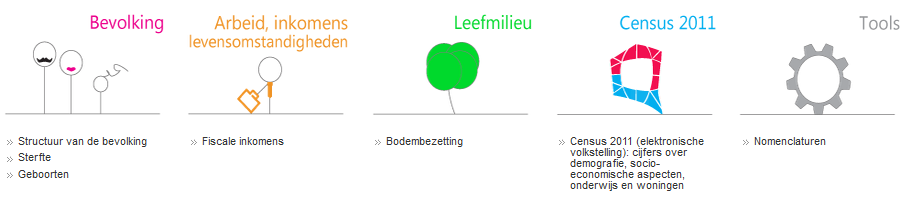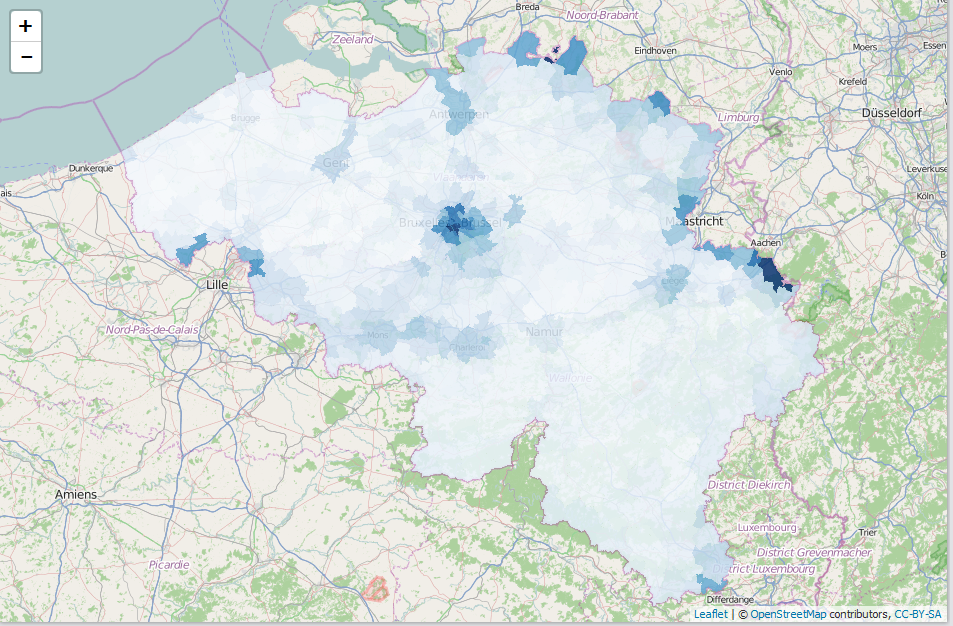# Open Data in Belgium - release of BelgiumStatistics R package

On 22/10/2015, the Belgium government launched its Open Data initiative by releasing a number of datasets related to population statistics, fiscal information, 'kadaster', the 2011 census and some tools. Because BNOSAC works a lot with these kind of data and because we like to promote open data, an R package called BelgiumStatistics was made available for R users at https://github.com/jwijffels/BelgiumStatisticsThe package contains all the datasets released by Statistics Belgium (Bevolking, Werk, Leefmilieu, Census 2011) under the 'Licentie open data'. Readily available to R users. Thanks to the open data, analysing and visualising Belgium data has now become a lot smoother as the example below shows.

```require(BelgiumStatistics)
require(data.table)
require(BelgiumMaps)
require(leaflet)
data(TF_SOC_POP_STRUCT_2015) ## Part of BelgiumStatistics
data(mapbelgium.fusiegemeenten.wgs) ## Part of BelgiumMaps (not released yet)

x <- as.data.table(TF_SOC_POP_STRUCT_2015)
x <- x[, list(MS_POPULATION = sum(MS_POPULATION),
Foreigners = sum(MS_POPULATION[TX_NATLTY_NL == "Vreemdelingen"]) / sum(MS_POPULATION),
Age = 100 * sum(MS_POPULATION * CD_AGE) / sum(MS_POPULATION),
Females = 100 * sum(MS_POPULATION[CD_SEX == "F"]) / sum(MS_POPULATION)),
by = list(CD_MUNTY_REFNIS, TX_MUNTY_DESCR_NL)]
x <- setDF(x)

mymap <- merge(mapbelgium.fusiegemeenten.wgs,
x, by.x = "ORDER08", by.y = "CD_MUNTY_REFNIS", all.x=TRUE, all.y=FALSE)
mymap <- subset(mymap, !is.na(Foreigners))
pal <- colorNumeric(palette = "Blues", domain = mymap\$Foreigners)
leaflet(mymap) %>%